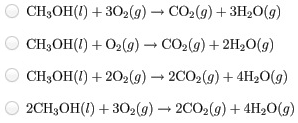# Problem: Write a balanced equation for the combustion of methanol.Calculate ΔH°rxn at 25°C.Calculate ΔS°rxn at 25°C.Calculate ΔG°rxn at 25°C.

###### FREE Expert Solution

We begin with the unbalanced combustion reaction of methanol:

CH3OH(l) + O2(g)  → CO2(g) + H2O(l)

Calculating the number of elements in reactants and products:

Reactants    Products

C         1                     1

H         4                     2

O         3                     3

79% (66 ratings)###### Problem Details

Write a balanced equation for the combustion of methanol.Calculate ΔH°rxn at 25°C.

Calculate ΔS°rxn at 25°C.

Calculate ΔG°rxn at 25°C.